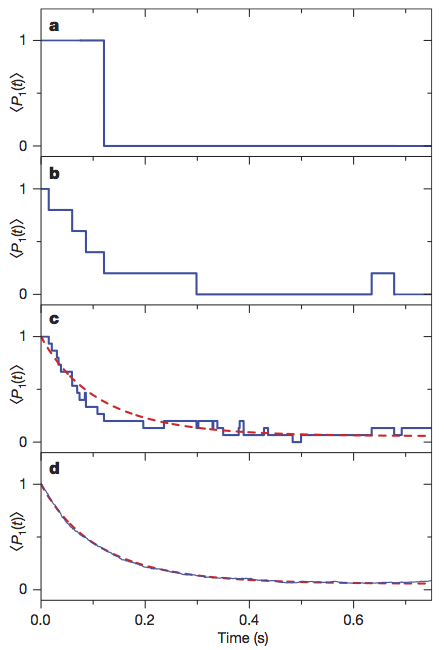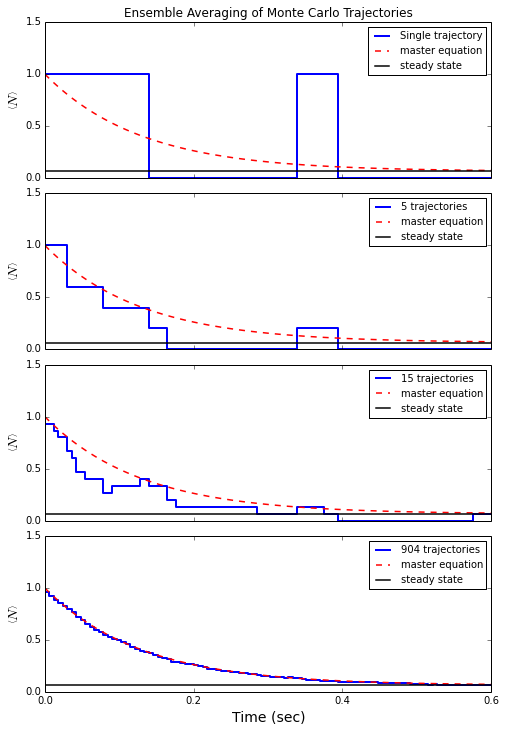# QuTiP lecture: Quantum Monte-Carlo Trajectories¶

Author: J. R. Johansson ([email protected]), http://dml.riken.jp/~rob/

The latest version of this IPython notebook lecture is available at http://github.com/jrjohansson/qutip-lectures.

The other notebooks in this lecture series are indexed at http://jrjohansson.github.com.

The example in this lecture is based on an example by P.D. Nation.

In :
%matplotlib inline
import matplotlib.pyplot as plt
import numpy as np

In :
from qutip import *


## Introduction to the Quantum Monte-Carlo trajectory method¶

The Quantum Monte-Carlo trajectory method is an equation of motion for a single realization of the state vector $\left|\psi(t)\right>$ for a quantum system that interacts with its environment. The dynamics of the wave function is given by the Schrodinger equation,

$\displaystyle\frac{d}{dt}\left|\psi(t)\right> = - \frac{i}{\hbar} H_{\rm eff} \left|\psi(t)\right>$

where the Hamiltonian is an effective Hamiltonian that, in addition to the system Hamiltonian $H(t)$, also contains a non-Hermitian contribution due to the interaction with the environment:

$\displaystyle H_{\rm eff}(t) = H(t) - \frac{i\hbar}{2}\sum_n c_n^\dagger c_n$

Since the effective Hamiltonian is non-Hermitian, the norm of the wavefunction is decreasing with time, which to first order in a small time step $\delta t$ is given by $\langle\psi(t+\delta t)|\psi(t+\delta t)\rangle \approx 1 - \delta p\;\;\;$, where

$\displaystyle \delta p = \delta t \sum_n \left<\psi(t)|c_n^\dagger c_n|\psi(t)\right>$

The decreasing norm is used to determine when so-called quantum jumps are to be imposed on the dynamics, where we compare $\delta p$ to a random number in the range [0, 1]. If the norm has decreased below the randomly chosen number, we apply a "quantum jump", so that the new wavefunction at $t+\delta t$ is given by

$\left|\psi(t+\delta t)\right> = c_n \left|\psi(t)\right>/\left<\psi(t)|c_n^\dagger c_n|\psi(t)\right>^{1/2}$

for a randomly chosen collapse operator $c_n$, weighted so the probability that the collapse being described by the nth collapse operator is given by

$\displaystyle P_n = \left<\psi(t)|c_n^\dagger c_n|\psi(t)\right>/{\delta p}$

## Decay of a single-photon Fock state in a cavity¶

This is a Monte-Carlo simulation showing the decay of a cavity Fock state $\left|1\right>$ in a thermal environment with an average occupation number of $n=0.063$ .

Here, the coupling strength is given by the inverse of the cavity ring-down time $T_c = 0.129$ .

The parameters chosen here correspond to those from S. Gleyzes, et al., Nature 446, 297 (2007), and we will carry out a simulation that corresponds to these experimental results from that paper:

In :
from IPython.display import Image
Image(filename='images/exdecay.png')

Out:### Problem parameters¶

In :
N = 4               # number of basis states to consider
kappa = 1.0/0.129   # coupling to heat bath
nth = 0.063         # temperature with <n>=0.063

tlist = np.linspace(0,0.6,100)


## Create operators, Hamiltonian and initial state¶

Here we create QuTiP Qobj representations of the operators and state that are involved in this problem.

In :
a = destroy(N)      # cavity destruction operator
H = a.dag() * a     # harmonic oscillator Hamiltonian
psi0 = basis(N,1)   # initial Fock state with one photon: |1>


## Create a list of collapse operators that describe the dissipation¶

In :
# collapse operator list
c_op_list = []

# decay operator
c_op_list.append(sqrt(kappa * (1 + nth)) * a)

# excitation operator
c_op_list.append(sqrt(kappa * nth) * a.dag())


## Monte-Carlo simulation¶

Here we start the Monte-Carlo simulation, and we request expectation values of photon number operators with 1, 5, 15, and 904 trajectories (compare with experimental results above).

In :
ntraj = [1, 5, 15, 904] # list of number of trajectories to avg. over

mc = mcsolve(H, psi0, tlist, c_op_list, [a.dag()*a], ntraj)

10.1%. Run time:   1.86s. Est. time left: 00:00:00:16
20.0%. Run time:   3.07s. Est. time left: 00:00:00:12
30.1%. Run time:   4.32s. Est. time left: 00:00:00:10
40.0%. Run time:   5.55s. Est. time left: 00:00:00:08
50.0%. Run time:   6.84s. Est. time left: 00:00:00:06
60.1%. Run time:   8.18s. Est. time left: 00:00:00:05
70.0%. Run time:   9.47s. Est. time left: 00:00:00:04
80.1%. Run time:  10.70s. Est. time left: 00:00:00:02
90.0%. Run time:  12.39s. Est. time left: 00:00:00:01
100.0%. Run time:  14.25s. Est. time left: 00:00:00:00
Total run time:  14.29s


The expectation values of $a^\dagger a$ are now available in array mc.expect[idx] where idx takes values in [0,1,2,3] corresponding to the averages of 1, 5, 15, 904 Monte Carlo trajectories, as specified above. Below we plot the array mc.expect[idx] vs. tlist for each index idx.

For comparison with the averages of single quantum trajectories provided by the Monte-Carlo solver we here also calculate the dynamics of the Lindblad master equation, which should agree with the Monte-Carlo simultions for infinite number of trajectories.

In :
# run master equation to get ensemble average expectation values
me = mesolve(H, psi0, tlist, c_op_list, [a.dag()*a])

# calulate final state using steadystate solver
fexpt = expect(a.dag()*a, final_state)  # find expectation value for particle number


## Plot the results¶

In :
import matplotlib.font_manager
leg_prop = matplotlib.font_manager.FontProperties(size=10)

fig, axes = plt.subplots(4, 1, sharex=True, figsize=(8,12))

fig.subplots_adjust(hspace=0.1) # reduce space between plots

for idx, n in enumerate(ntraj):

axes[idx].step(tlist, mc.expect[idx], 'b', lw=2)
axes[idx].plot(tlist, me.expect, 'r--', lw=1.5)
axes[idx].axhline(y=fexpt, color='k', lw=1.5)

axes[idx].set_yticks(np.linspace(0, 2, 5))
axes[idx].set_ylim([0, 1.5])
axes[idx].set_ylabel(r'$\left<N\right>$', fontsize=14)

if idx == 0:
axes[idx].set_title("Ensemble Averaging of Monte Carlo Trajectories")
axes[idx].legend(('Single trajectory', 'master equation', 'steady state'), prop=leg_prop)
else:
axes[idx].legend(('%d trajectories' % n, 'master equation', 'steady state'), prop=leg_prop)

axes.xaxis.set_major_locator(plt.MaxNLocator(4))
axes.set_xlabel('Time (sec)',fontsize=14);### Software versions:¶

In :
from qutip.ipynbtools import version_table

version_table()

Out:
SoftwareVersion
QuTiP3.0.0.dev-5a88aa8
Numpy1.8.1
Python3.4.1 (default, Jun 9 2014, 17:34:49) [GCC 4.8.3]
IPython2.0.0
SciPy0.13.3
matplotlib1.3.1
Cython0.20.1post0
OSposix [linux]
Thu Jun 26 15:01:46 2014 JST